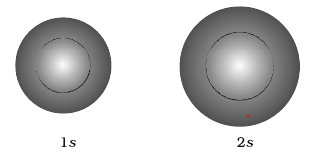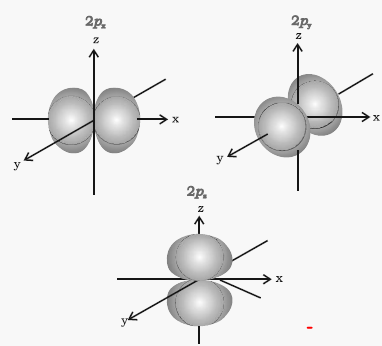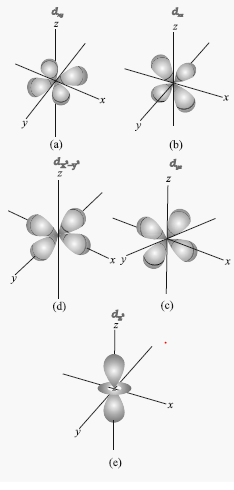# Shapes of Atomic Orbitals:Boundary Surface Diagrams & Nodes

## Shapes of Orbitals

### Probability Density

ψ gives us the amplitude of wave. The value of ψ has no physical significance.
|Ψ|2 gives us the region in which the probability of finding an electron is maximum. It is called probability density.

### Nodal surfaces

The region where this probability density function reduces to zero is called nodal surfaces or simply nodes.
There are two types of Nodes

#### Radial nodes or Nodal Region

Radial nodes occur when the probability density of wave function for the electron is zero on a spherical surface of a radius. Number of radial nodes = n – l – 1

#### Angular nodes or Nodal Planes

Angular nodes occur when the probability density wave function for the electron is zero along the directions specified by a particular angle. Number of angular nodes = l
Therefore ,
Number of angular nodes =l
Total number of nodes =Number of radial nodes + Number of angular nodes= n – 1
Example
2s orbital has n=2 and l=0. The number of angular nodes = l = 0. The number of radial nodes = [(n-1) - l] = [1 - 0] = 1. Total Number of Nodes=1
2p orbital has n=2 and l=1. The number of angular nodes = l = 1. The number of radial nodes = [(n-1) - l] = [1 - 1] = 0. Total Number of Nodes=1

### Boundary Surface Diagrams

It is surface in the space where probability density is constant for a given orbital. This gives  a good representation of the shape of the orbital. This shapes encloses the volume or region where probability of finding electron is high

Shape of S-orbital
• All the s -orbital are Spherical shape
• The probability of finding the electron at a given distance is equal in all the directions.
• The size of the s orbital increases with increase in n, that is, 4s > 3s > 2s > 1s and the electron is located further away from the nucleus as the principal quantum number increases.Shape of P orbitals
• It has 3 possible orientation
• Each p orbital consists of two sections called lobes that are on either side of the plane that passes through the nucleus
• The probability density function is zero on the plane where the two lobes touch each other.
• The size, shape and energy of the three orbitals are same just the orientation is different
• They are given the designations $2p_x$, $2p_y$, and $2p_z$Shape of D Orbitals
• It has five orientations
• The shapes of the first four D orbitals are similar to each other, where as that of the fifth one is different from others, but all five 3d orbitals are equivalent in energy
• The five d-orbitals are designated as $d_{xy}$, $d_{yz}$,$d_{xz}$,$d_{x^2 - y^2}$ and $d_{z^2}$.Question 1
Which orbital is dumb-bell shaped
(a) s-orbital
(b) p-orbital
(c) d-orbital
(d) f-orbital
Solution
(b)

Question 2
For the dumb-bell shaped orbital, the value of l is
(a) 3
(b) 0
(c) 1
(d) 2
Solution
(c)

Question 3
Which of the sub-shell is circular
(a) 4s
(b) 4f
(c) 4p
(d) 4d
Solution
(a)

Question 4
The shape of $d_{xy}$ orbital will be
(a) Circular
(b) Dumb-bell
(c) Double dumb-bell
(d) Trigonal
Solution
(c)

Question 5
Number of nodal centres for 2s orbital
(a) 1
(b) 0
(c) 4
(d) 3
Solution
(a)